VeighNa量化社区

### 数据分析的必要性

1. 首先，数据质量的好坏决定策略实盘的可行性，例如下载仿真历史数据，却用来研究实盘策略，就会导致典型的“Garbage in, garbage out”。
2. 其次，下载的历史数据不应该出现某段时间缺失的情况，这就要求建模分析的第一步是对行情数据进行画图，通过人眼初步判断数据的好坏质量：即无缺失的行情部分、也无明显的“异常”数据点。
3. 确定数据质量可靠后，就可以分析数据特性，从而决定其适用于开发哪种类型的策略。若数据自相关性弱，属于平稳时间序列，符合正态分布，可以考虑用作统计套利；若数据自相关性强，且非平稳时间序列，有明显的肥尾特征，则可以用来开发趋势跟踪类的策略（CTA）。

1. 对行情数据画图，确定无断点；
2. 进行随机性检验，确定数据具有非纯随机性；
3. 进行平稳性检验，确定时间序列的不平稳性；
4. 画出自相关图，确定时间序列自相关性强；
5. 相对波动率分析，确定其平均波动率大于固定交易成本（手续费）；
6. 行情变化率分析，确定其符合肥尾分布；
7. 计算相关计算指标（DataFrame格式），可以与行情图对比分析；
8. 合成更高维度的K线，重复前面1~7步。

### 数据分析流程

• symbol：合约代码，由用户定义，字符串格式;
• exchange：交易所代码，Exchange枚举值格式;
• interval：K线周期，Interval枚举值格式，如Interval.MINUTE;
• start：数据开始时间，datetime格式，如datetime(2019, 4, 1)；
• end：数据结束时间，datetiem格式，如datetime(2019, 10, 30)；

``````from vnpy.trader.database import database_manager

output("开始加载历史数据")
symbol=symbol,
exchange=exchange,
interval=interval,
start=start,
end=end,
)
output(f"历史数据加载完成，数据量：{len(bars)}")

def output(msg):
""""""
print(f"{datetime.now()}\t{msg}")``````

``````# Generate history data in DataFrame
t = []
o = []
h = []
l = []
c = []

for bar in bars:
time = bar.datetime
open_price = bar.open_price
high_price = bar.high_price
low_price = bar.low_price
close_price = bar.close_price

t.append(time)
o.append(open_price)
h.append(high_price)
l.append(low_price)
c.append(close_price)

self.orignal = pd.DataFrame()
self.orignal["open"] = o
self.orignal["high"] = h
self.orignal["low"] = l
self.orignal["close"] = c
self.orignal.index = t``````

``````output("第一步:画出行情图，检查数据断点")
self.orignal["close"].plot(figsize=(20, 8), title="close_price")
plt.show()``````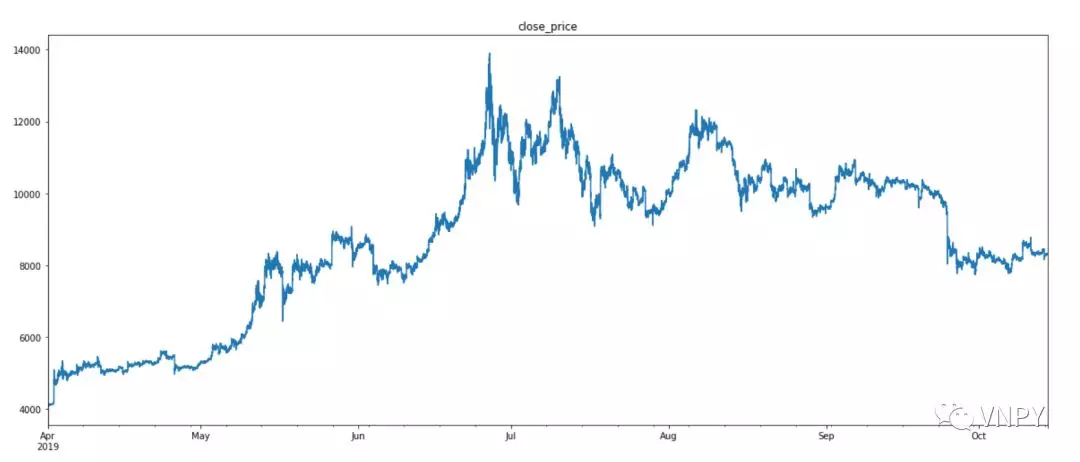• 若p值>0.05，证明数据具有纯随机性：不具备某些数据特征，没有数据分析意义；
• 若p值<0.05，证明数据不具有纯随机性：具备某些数据特性，进行数据分析有利于开发出适应其统计规律的交易策略。

``````from statsmodels.stats.diagnostic import acorr_ljungbox

def random_test(close_price):
"""
白噪声检验
"""
acorr_result = acorr_ljungbox(close_price, lags=1)
p_value = acorr_result
if p_value < 0.05:
output("第二步：随机性检验：非纯随机性")
else:
output("第二步：随机性检验：纯随机性")
output(f"白噪声检验结果:{acorr_result}\n")``````CTA策略研究的是非平稳性时间序列，平稳时间序列适用于期货价差的统计套利。

``````from statsmodels.tsa.stattools import adfuller as ADF

def stability_test(close_price):
"""
平稳性检验
"""
t_s = statitstic
t_c = statitstic["10%"]

if t_s > t_c:
output("第三步：平稳性检验：存在单位根，时间序列不平稳")
else:
output("第三步：平稳性检验：不存在单位根，时间序列平稳")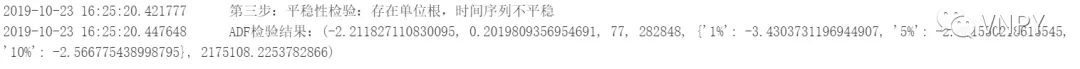• 自相关图：统计相关性总结了两个变量之间的关系强度，即描述了一个观测值与另一个观测值之间的自相关，包括直接和间接的相关性信息。这种关系的惯性将继续到之后的滞后值，随着效应被削弱而在某个点上缩小到没有。
• 偏自相关图：偏自相关是剔除干扰后时间序列观察与先前时间步长时间序列观察之间关系的总结，即只描述观测值与其滞后之间的直接关系。可能超过k的滞后值不会再有相关性。

``````from statsmodels.graphics.tsaplots import plot_acf, plot_pacf

def autocorrelation_test(close_price):
"""
自相关性检验
"""
output("第四步：画出自相关性图，观察自相关特性")

plot_acf(close_price, lags=60)
plt.show()

plot_pacf(close_price, lags=60).show()
plt.show()``````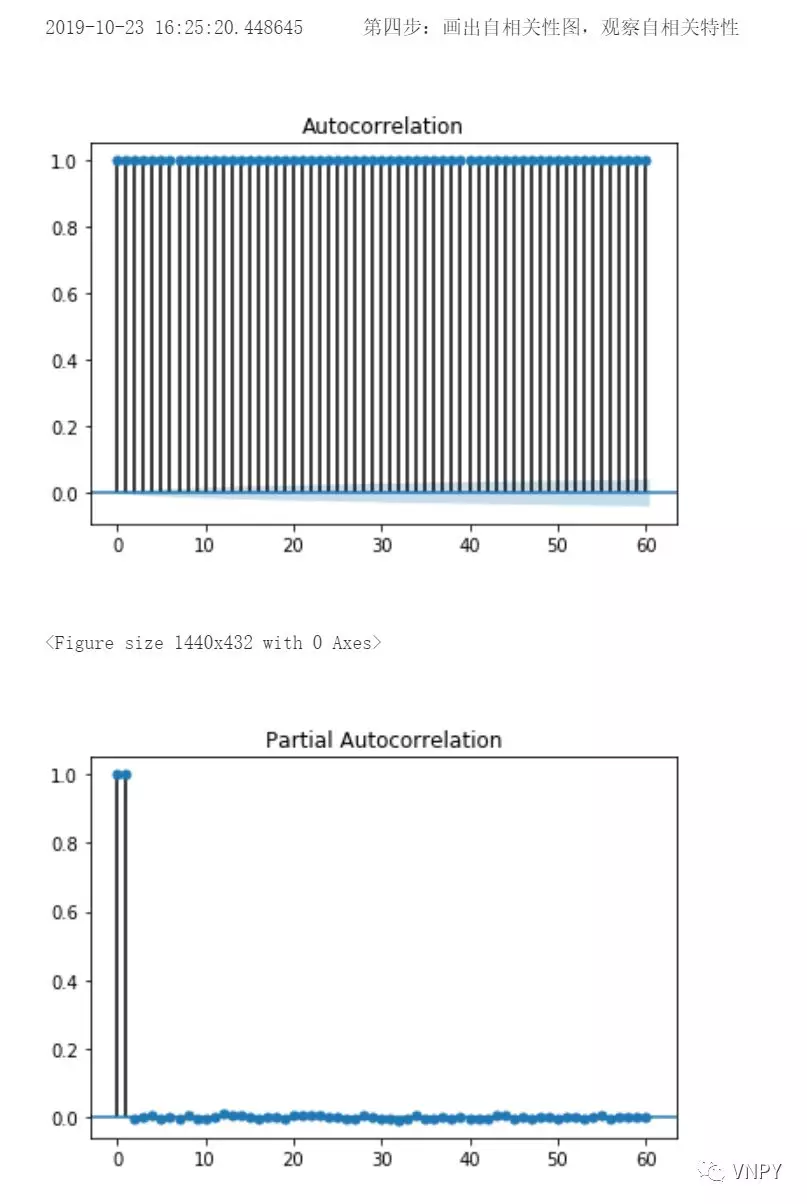1. 调用talib库的ATR函数计算1分钟K线的绝对波动率；
2. 通过收盘价*手续费计算固定成本；
3. 相对波动率=绝对波动率-固定成本；
4. 对相对波动率进行画图：时间序列图和频率分布图；
5. 对相对波动率进行描述统计分析，得到平均数、中位数、偏度、峰度

``````def relative_volatility_analysis(self, df: DataFrame = None):
"""
相对波动率
"""
output("第五步：相对波动率分析")
df["volatility"] = talib.ATR(
np.array(df['high']),
np.array(df['low']),
np.array(df['close']),
self.window_volatility
)

df["fixed_cost"] = df["close"] * self.rate
df["relative_vol"] = df["volatility"] - df["fixed_cost"]

df["relative_vol"].plot(figsize=(20, 6), title="relative volatility")
plt.show()

df["relative_vol"].hist(bins=200, figsize=(20, 6), grid=False)
plt.show()

statitstic_info(df["relative_vol"])

def statitstic_info(df):
"""
描述统计信息
"""
mean = round(df.mean(), 4)
median = round(df.median(), 4)
output(f"样本平均数：{mean}, 中位数: {median}")

skew = round(df.skew(), 4)
kurt = round(df.kurt(), 4)

if skew == 0:
skew_attribute = "对称分布"
elif skew > 0:
skew_attribute = "分布偏左"
else:
skew_attribute = "分布偏右"

if kurt == 0:
kurt_attribute = "正态分布"
elif kurt > 0:
kurt_attribute = "分布陡峭"
else:
kurt_attribute = "分布平缓"

output(f"偏度为：{skew}，属于{skew_attribute}；峰度为：{kurt}，属于{kur
t_attribute}
\n")``````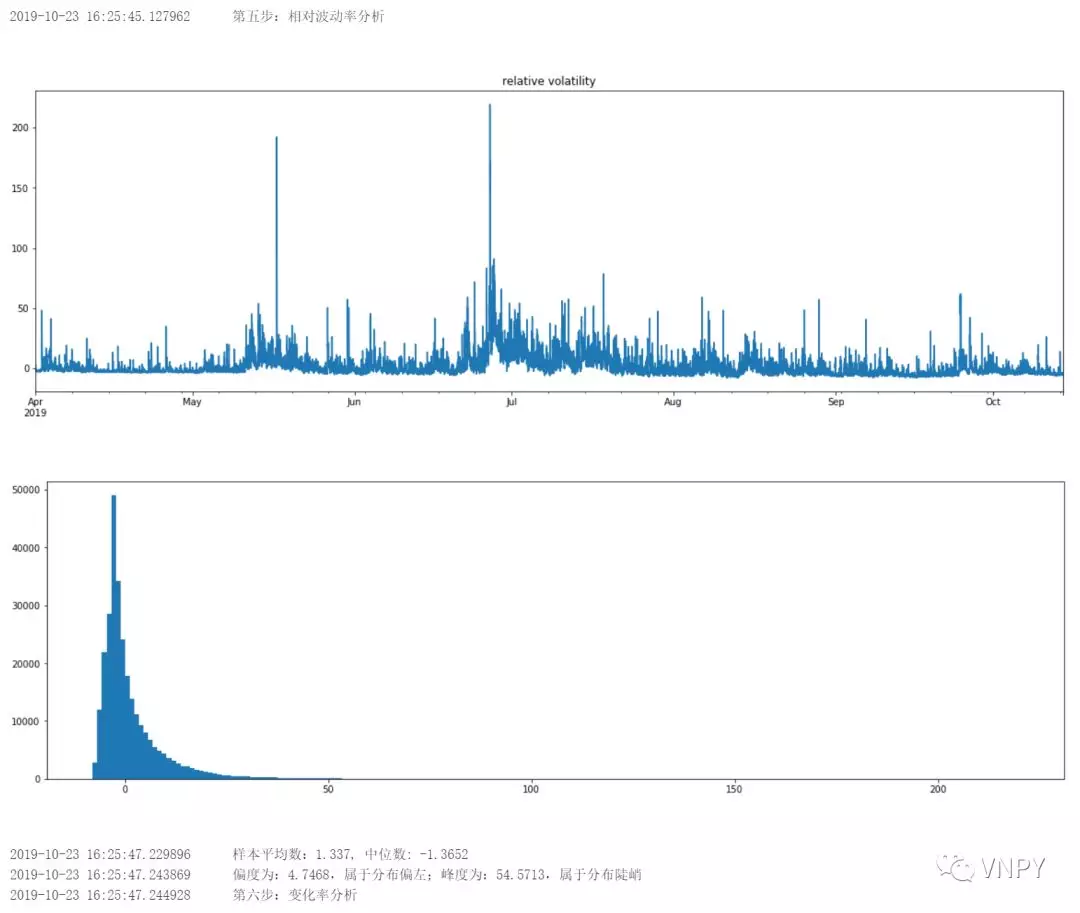1. 计算百分比变化率 = 100*（收盘价- 上一根收盘价）/上一根收盘价；
2. 对变化率进行画图：时间序列图和频率分布图；
3. 对变化率进行描述统计分析，得到平均数、中位数、偏度、峰度。

``````def growth_analysis(self, df: DataFrame = None):
"""
百分比K线变化率
"""
output("第六步：变化率分析")
df["pre_close"] = df["close"].shift(1).fillna(0)
df["g%"] = 100 * (df["close"] - df["pre_close"]) / df["close"]

df["g%"].plot(figsize=(20, 6), title="growth", ylim=(-5, 5))
plt.show()

df["g%"].hist(bins=200, figsize=(20, 6), grid=False)
plt.show()

statitstic_info(df["g%"])``````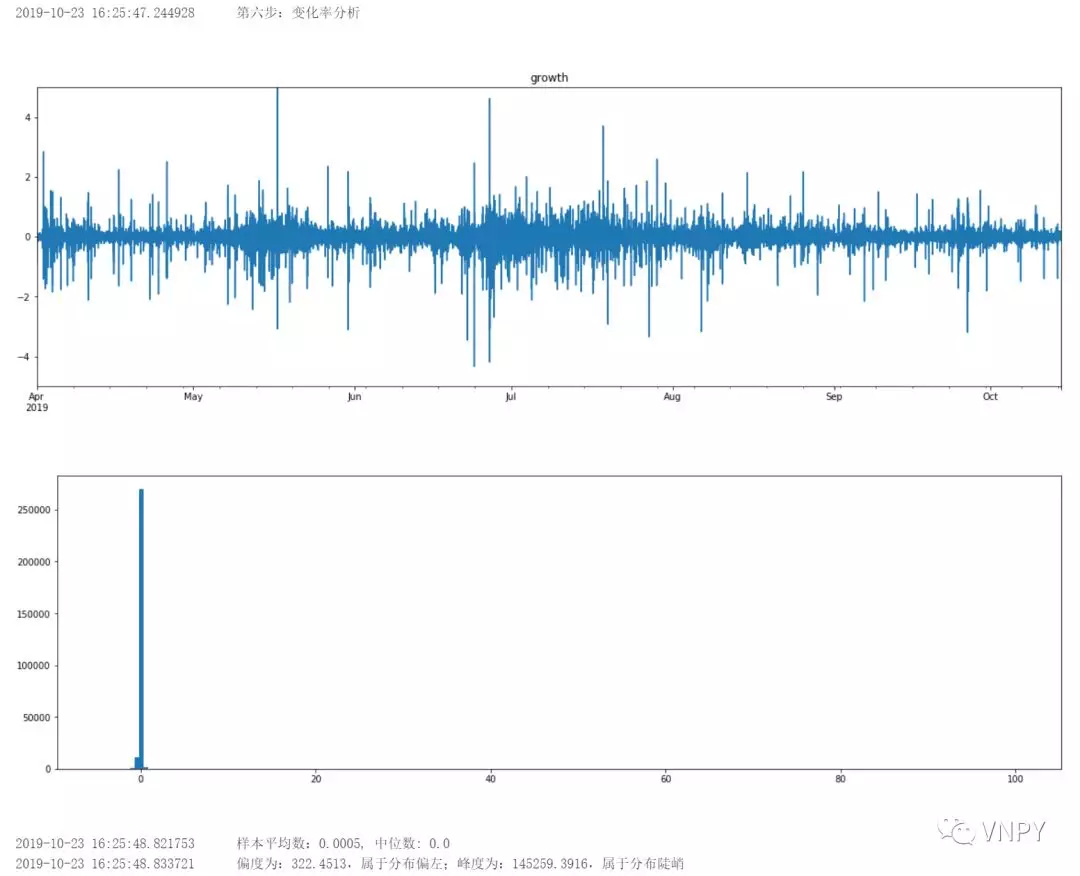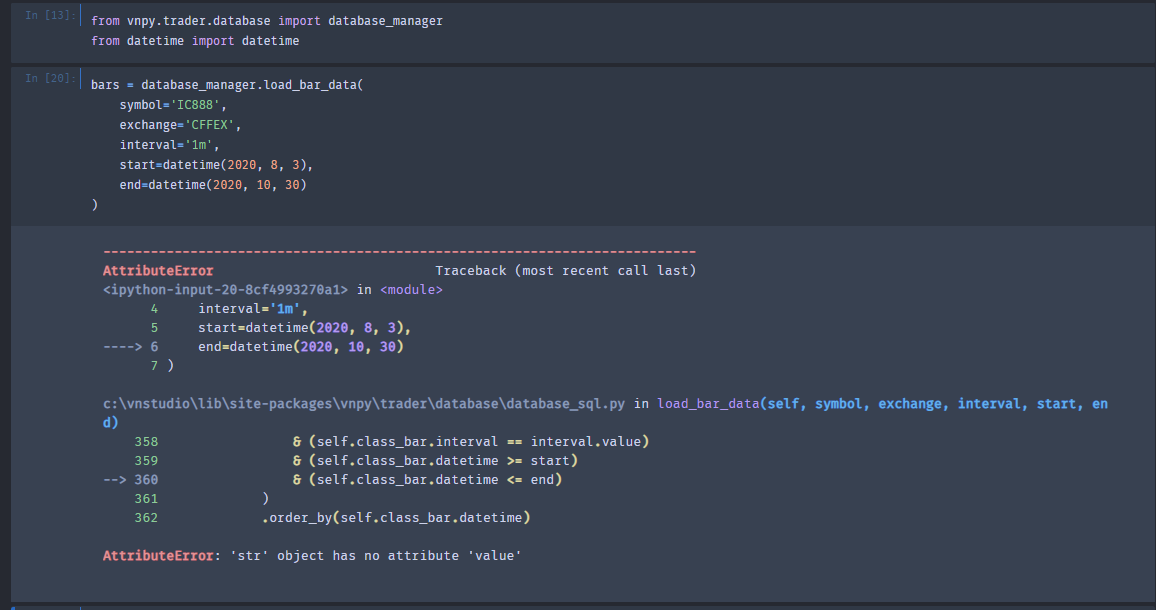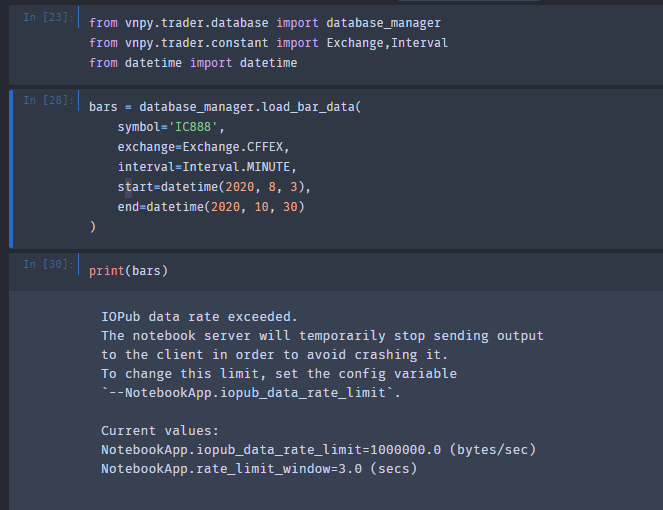from vnpy.trader.database import database_manager 提示database_manager 没有定义？

``````from vnpy.trader.database import get_database

database_manager = get_database()``````